Question

# 4) Air at 101 kPa and 360 K flows at 15 m/s over a flat plate...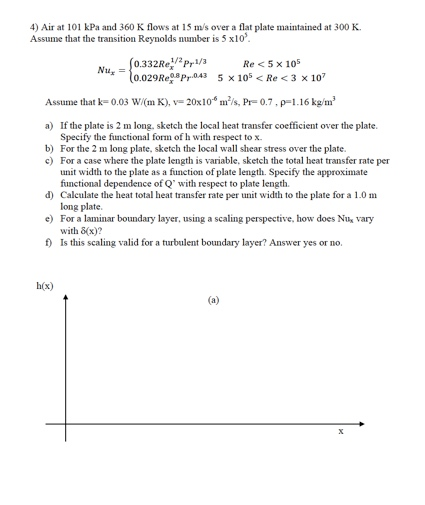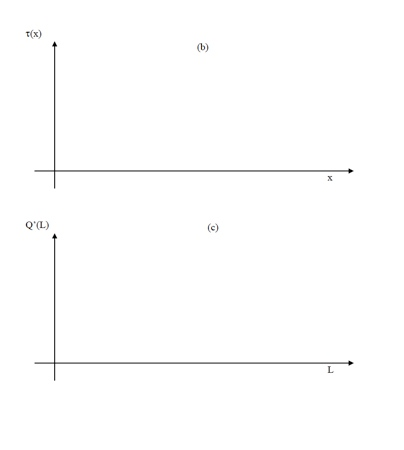4) Air at 101 kPa and 360 K flows at 15 m/s over a flat plate maintained at 300 K Assume that the transition Reynolds number is 5x10 (0.332R 12Pr1/3 Re < 5 x 105 0.029Repr0:43 5 x 105 < Re <3 x 107 Assume that k-0.03 W/( mK), 20x10* m?ls, Pr-0.7.p=1.16 kg/m a) If the plate is 2 m long, sketch the local heat transfer coefficient over the plate. Specify the functional form of h with respect tox b) For the 2 m long plate, sketch the local wall shear stress over the plate. c) For a case where the plate length is variable, sketch the total heat transfer rate per unit width to the plate as a function of plate length. Specify the approximate functional dependence of Q' with respect to plate length d) Calculate the heat total heat transfer rate per unit width to the plate for a 1.0 m long plate e) For a laminar boundary layer, using a scaling perspective, how does Nu, vary with 8x)? 1) Is this scaling valid for a turbulent boundary layer? Answer yes or no. h(x)
We were unable to transcribe this image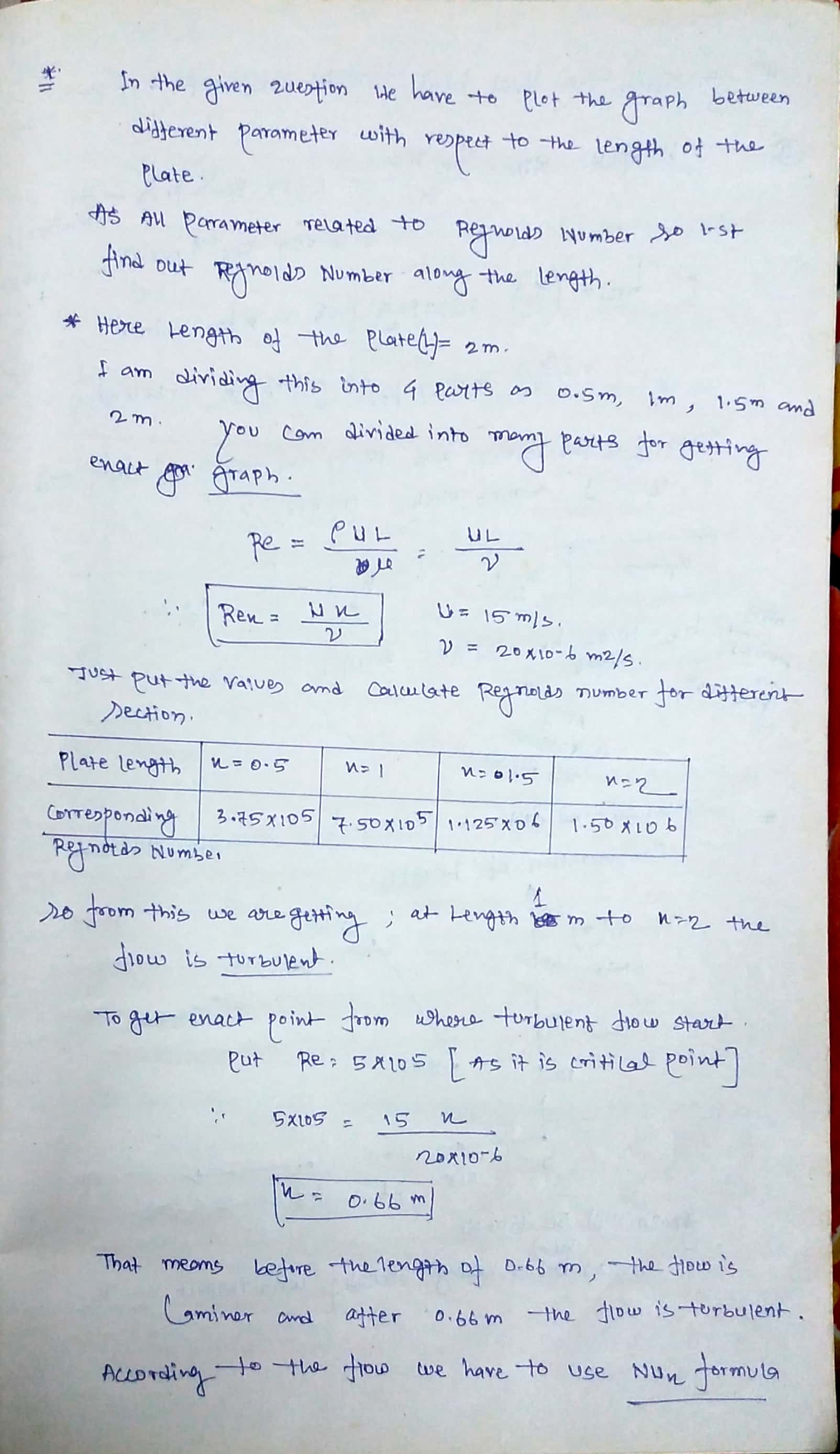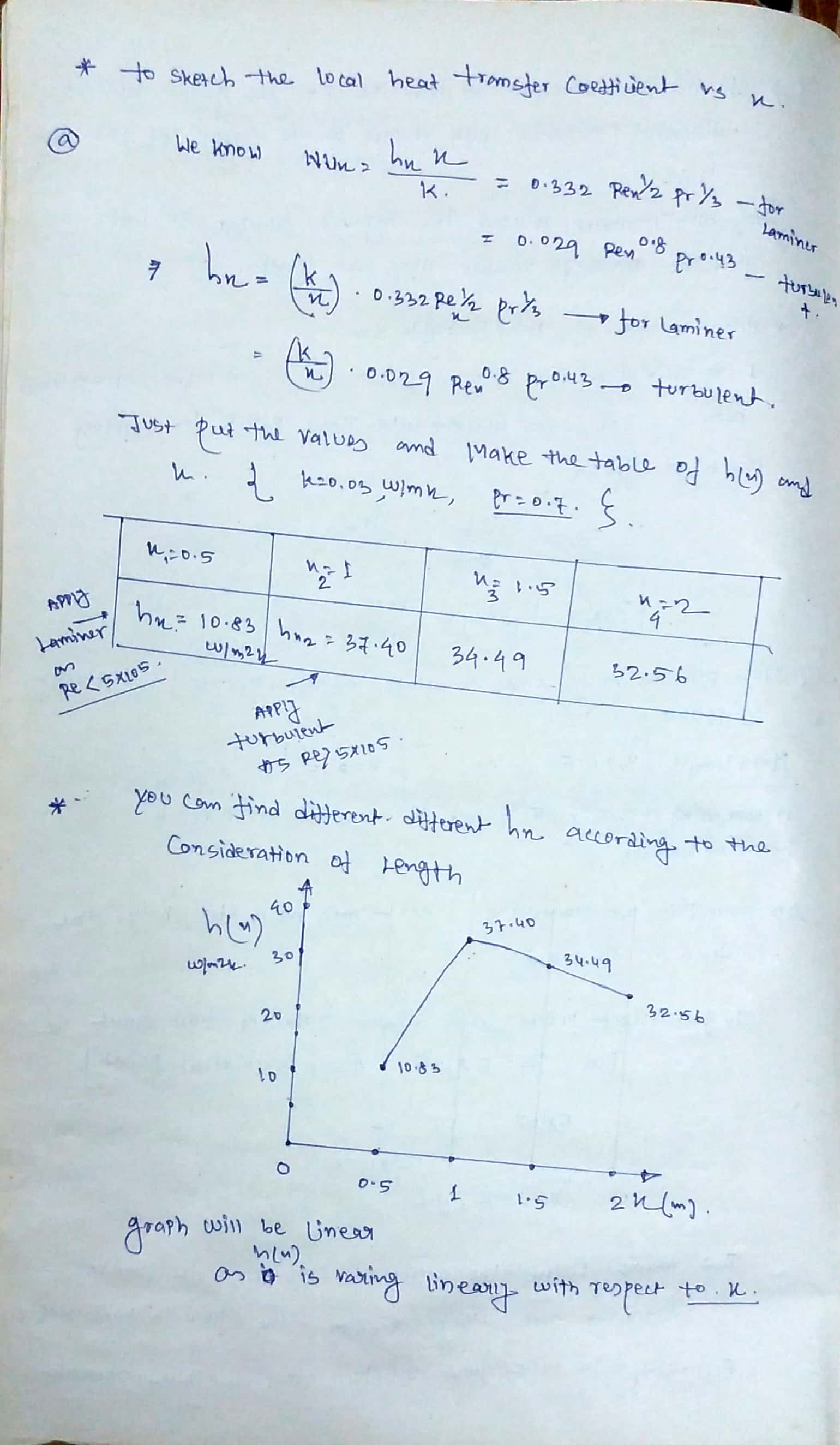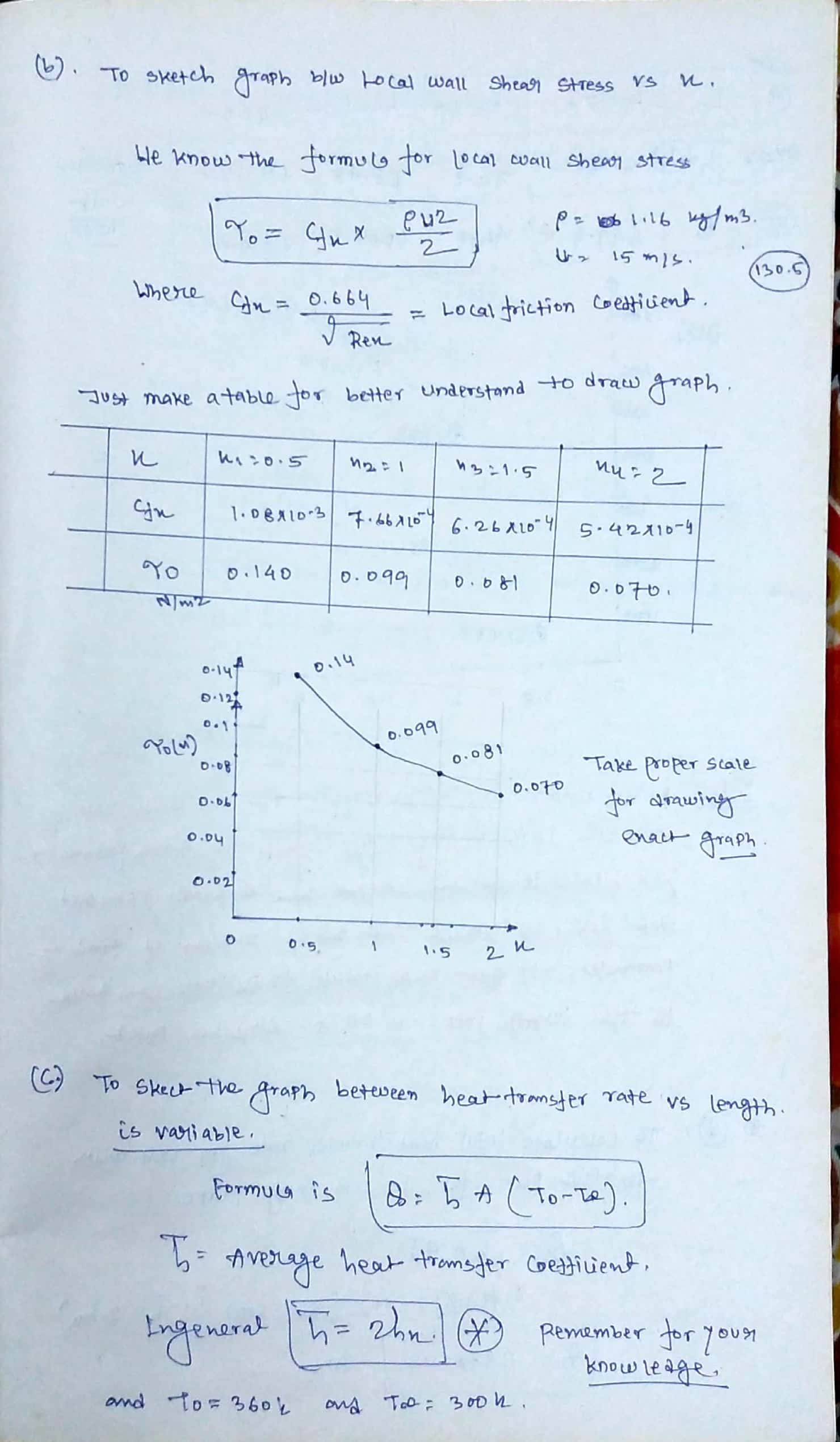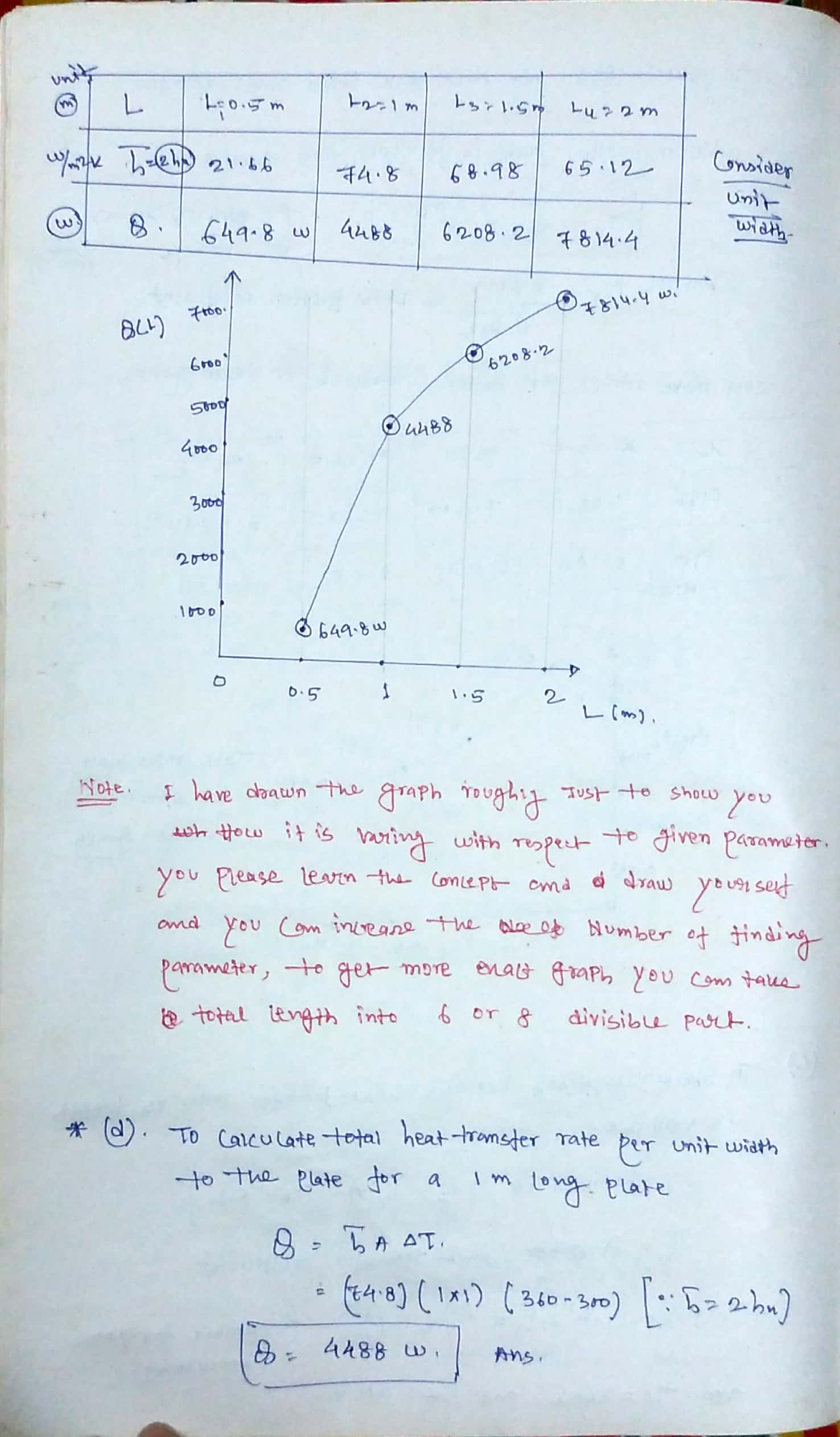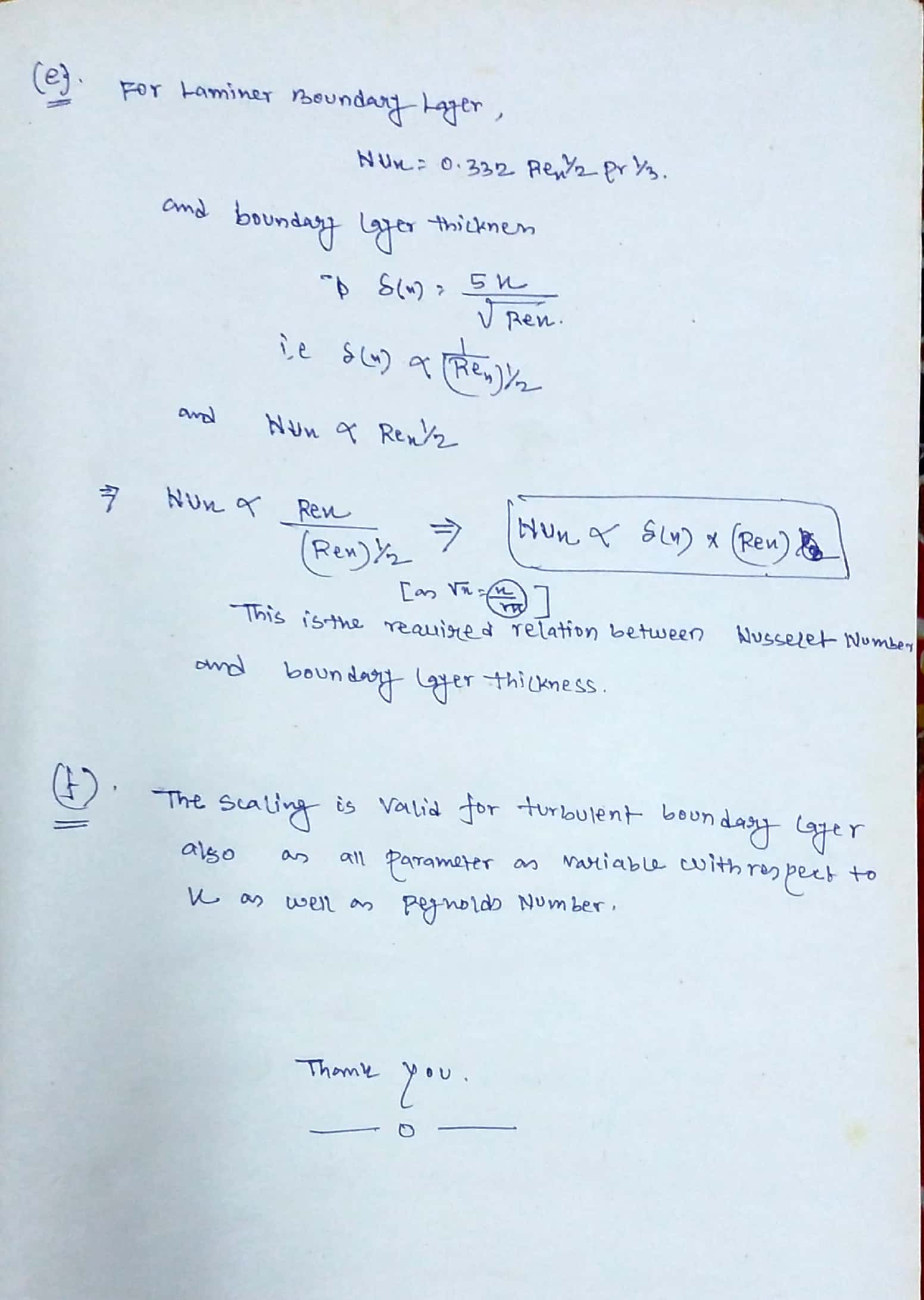#### Earn Coins

Coins can be redeemed for fabulous gifts.

Similar Homework Help Questions
• ### Air at a temperature of 300 K flows over one side of a flat plate of...

Air at a temperature of 300 K flows over one side of a flat plate of width 1 m at a velocity of 20 m/s. The plate has a constant surface temperature of 350 K. Assume Re(x,c)=5x10^5. a) What is the velocity boundary layer thickness at the end of the plate if L=0.25 m? What if L=1 m? b) Calculate the drag on the plate if L=0.25 m. What is the drag if L=1 m? c) Find the heat transfer...

• ### Exercise 2 Air at 20 °C and 1 atm flows over a flat plate at 50...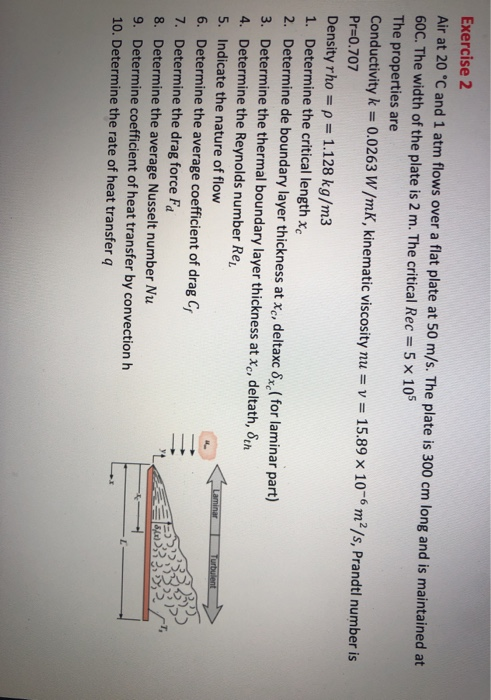Exercise 2 Air at 20 °C and 1 atm flows over a flat plate at 50 m/s. The plate is 300 cm long and is maintained at 60C. The width of the plate is 2 m. The critical Rec = 5 x 105 The properties are Conductivity k = 0.0263 W/mK, kinematic viscosity nu = v = 15.89 x 10-6 m²/s, Prandtl number is Pr=0.707 Density rho = p = 1.128 kg/m3 1. Determine the critical length Xc 2. Determine...

• ### 8) Air flows over a flat plate at a velocity of 20 m/s and a temperature...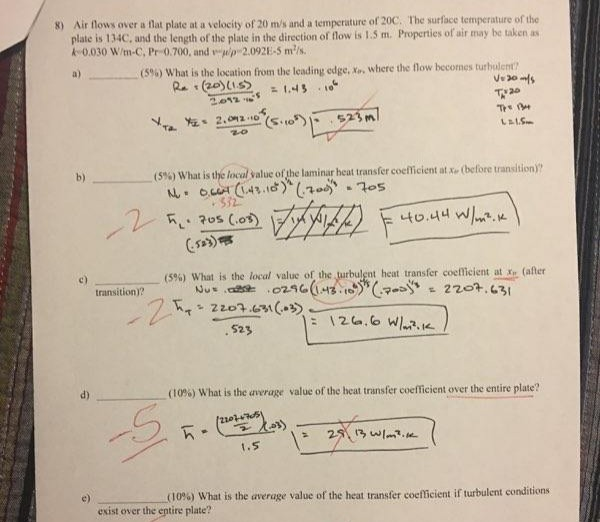8) Air flows over a flat plate at a velocity of 20 m/s and a temperature of 20C. The surface temperature of the plate is 134C, and the length of the plate in the direction of flow is 1.5 m. Properties of air may be taken as A-0.030 W/m-C, Pr-0.700, and a/p 2.092E-5 m/s (5% ) What is the location from the leading edge, X, where the flow becomes turbulent Re (20)15 a l Ve30s T20 T LaLS 523 m...

• ### Air flows with a velocity of 20 m/s over a flat plate of length 2 m....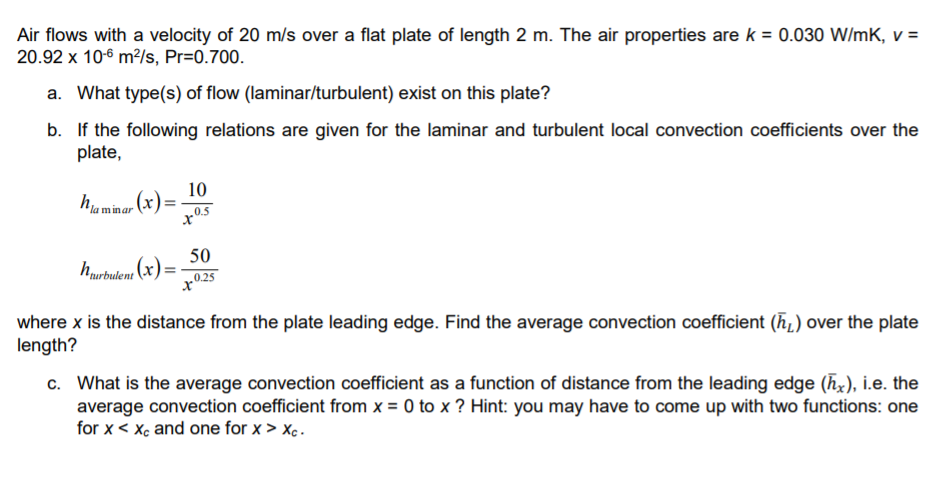Air flows with a velocity of 20 m/s over a flat plate of length 2 m. The air properties are k = 0.030 W/mK, V = 20.92 x 10-6 m/s, Pr=0.700. a. What type(s) of flow (laminar/turbulent) exist on this plate? b. If the following relations are given for the laminar and turbulent local convection coefficients over the plate, haminar (x) = 20 houwbudemo (x) = .52 where x is the distance from the plate leading edge. Find the average...

• ### Consider air flows with velocity of U?=U= 10 m/s over a semi-finite smooth flat plate with...Consider air flows with velocity of U?=U= 10 m/s over a semi-finite smooth flat plate with L=97 cm long. Calculate the followings by assuming ? = 1.568 x 10-5 m2/s and ?=1.177 kg/m3. Figure 1 : Boundary layer over a flat plate Consider air flows with velocity of U?=U=10 m/s over a semi-finite smooth flat plate with L=97 cm long. Calculate the followings by assuming ? = 1.568 x 10-5 m2/s and ?=1.177 kg/m3. b) Under some flow and boundary...

• ### 2. (25 pts) Engine oil flows with a velocity of 6 m/s over a flat plate...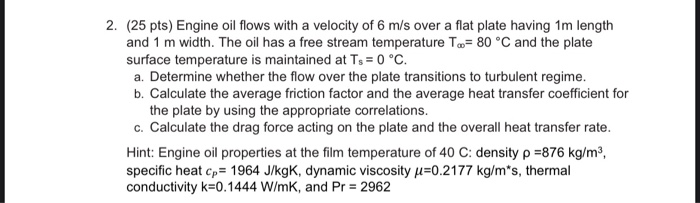2. (25 pts) Engine oil flows with a velocity of 6 m/s over a flat plate having 1m length and 1 m width. The oil has a free stream temperature To= 80 °C and the plate surface temperature is maintained at Ts = 0 °C. a. Determine whether the flow over the plate transitions to turbulent regime. b. Calculate the average friction factor and the average heat transfer coefficient for the plate by using the appropriate correlations. c. Calculate the...

• ### Air at 25 °C and 1 atm is flowing over a long flat plate with a...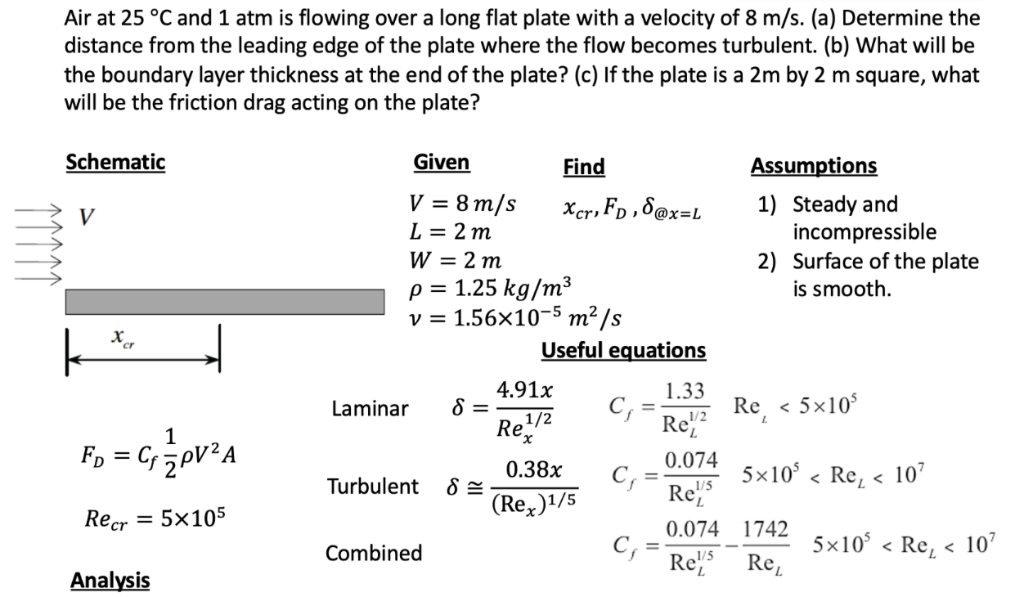Air at 25 °C and 1 atm is flowing over a long flat plate with a velocity of 8 m/s. (a) Determine the distance from the leading edge of the plate where the flow becomes turbulent. (b) What will be the boundary layer thickness at the end of the plate? (c) If the plate is a 2m by 2 m square, what will be the friction drag acting on the plate? Schematic Given ssumptions Find v=8m/s xcr ,FD ,δ@x=L L-2m...

• ### RCES Problem 7.002 Engine oil at 100°C and a velocity of 0.20 m/s flows over both...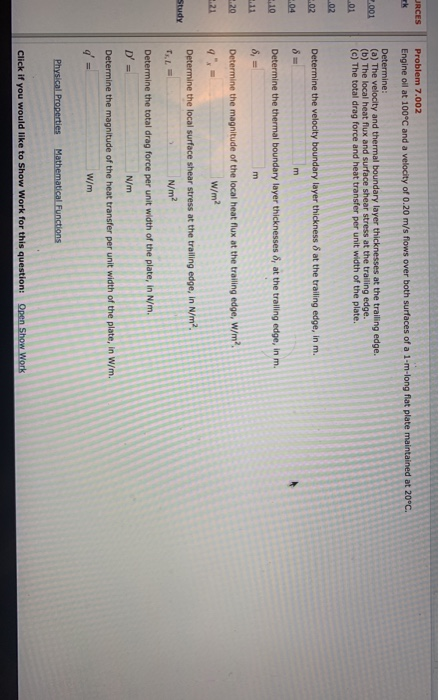RCES Problem 7.002 Engine oil at 100°C and a velocity of 0.20 m/s flows over both surfaces of a 1-m-long flat plate maintained at 20°C 001 Determine: (a) The velocity and thermal boundary layer thicknesses at the trailing edge. (b) The local heat flux and surface shear stress at the trailing edge. (c) The total drag force and heat transfer per unit width of the plate. 02 Determine the velocity boundary layer thickness 6 at the trailing edge, in m....

• ### Problem #3 Air flows over a flat plate at 4 m/s. An approximation for the x...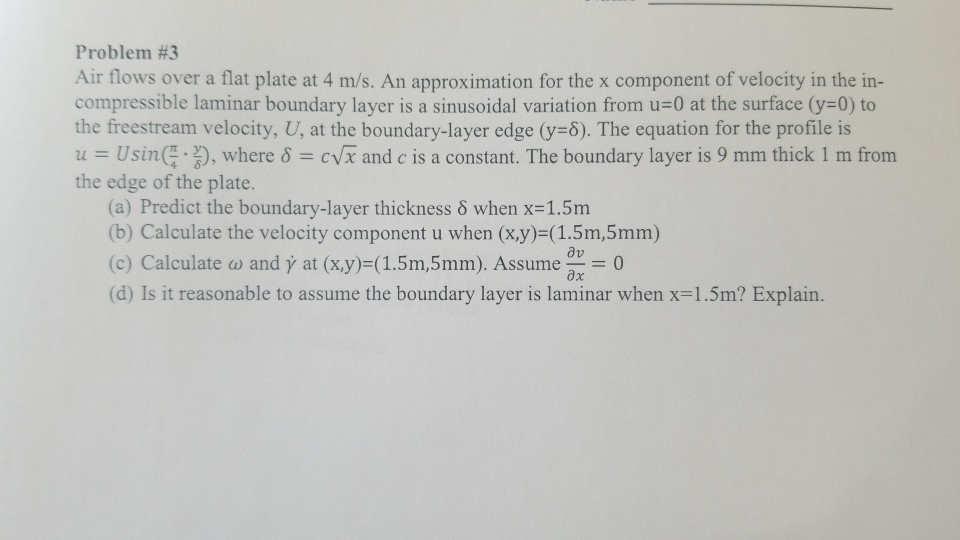Problem #3 Air flows over a flat plate at 4 m/s. An approximation for the x component of velocity in the in- compressible laminar boundary layer is a sinusoidal variation from u-0 at the surface (y-0) to the freestream velocity, U, at the boundary-layer edge (y-5). The equation for the profile is u-Usin( %), where cVx and c is a constant. The boundary layer is 9 mm thick 1 m from the edge of the plate. (a) Predict the boundary-layer...

• ### Problem 1: Atmospheric air at 25°C flows over both surfaces of 2 m long flat plate...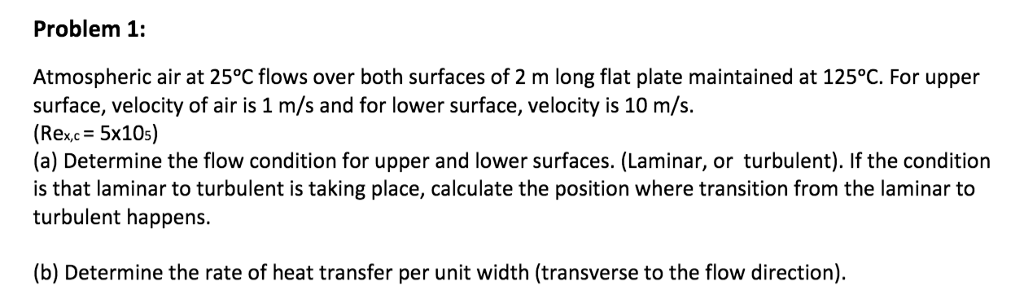Problem 1: Atmospheric air at 25°C flows over both surfaces of 2 m long flat plate maintained at 125°C. For upper surface, velocity of air is 1 m/s and for lower surface, velocity is 10 m/s. (Rex,c = 5x105) (a) Determine the flow condition for upper and lower surfaces. (Laminar, or turbulent). If the condition is that laminar to turbulent is taking place, calculate the position where transition from the laminar to turbulent happens. (b) Determine the rate of heat...